# Gear Ratio and Power Transfer 1 Gear Ratio

• Slides: 11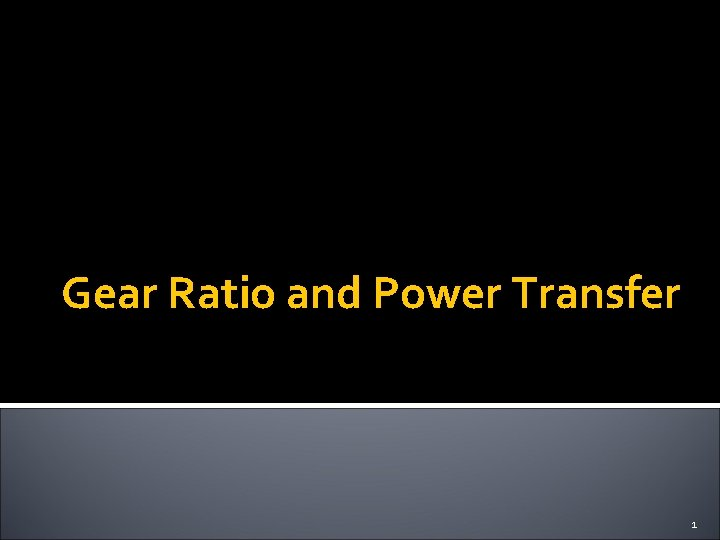Gear Ratio and Power Transfer 1Gear Ratio Gear ratio is the numerical relationship between the input and output of a gearbox. Input Gear: 20 Teeth Output Gear: 40 Teeth The picture shows a 20 tooth input that will turn twice when the 40 tooth output turns once This is a 2: 1 gear ratio 2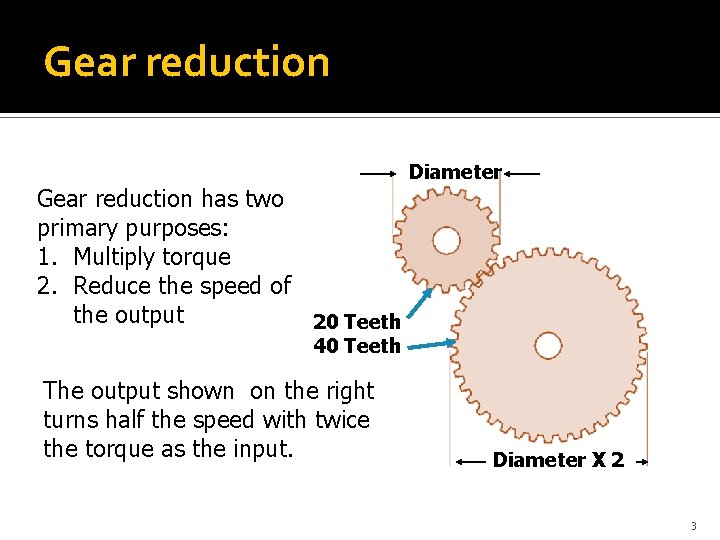Gear reduction has two primary purposes: 1. Multiply torque 2. Reduce the speed of the output 20 Teeth Diameter 40 Teeth The output shown on the right turns half the speed with twice the torque as the input. Diameter X 2 3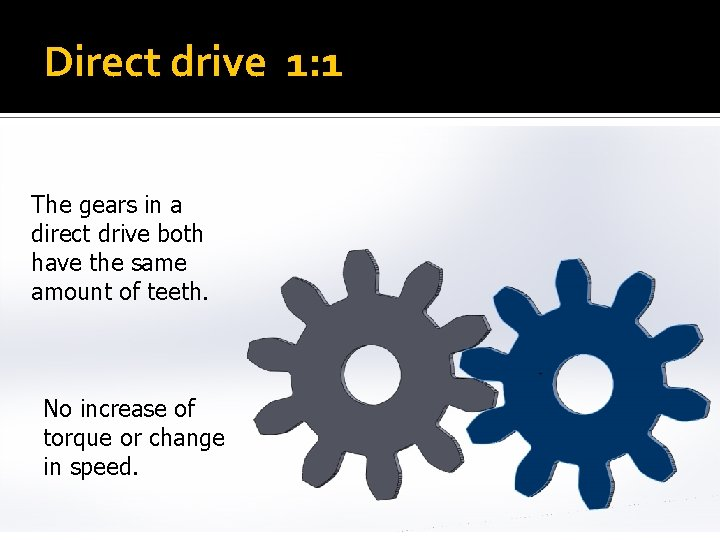Direct drive 1: 1 The gears in a direct drive both have the same amount of teeth. No increase of torque or change in speed. 4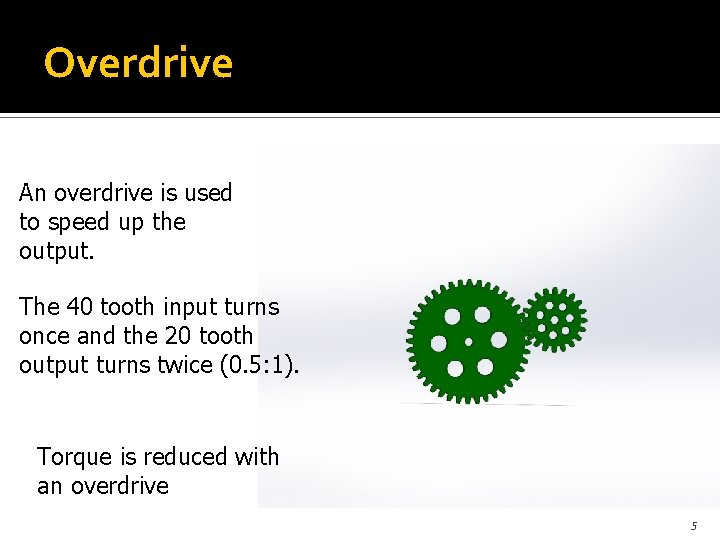Overdrive An overdrive is used to speed up the output. The 40 tooth input turns once and the 20 tooth output turns twice (0. 5: 1). Torque is reduced with an overdrive 5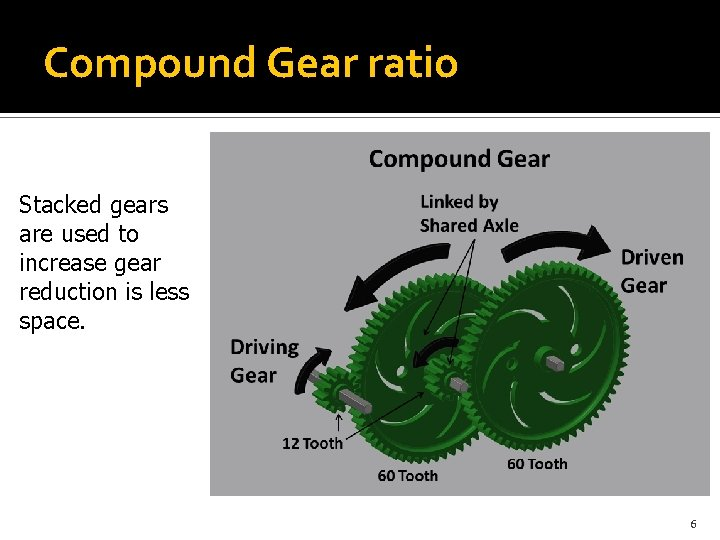Compound Gear ratio Stacked gears are used to increase gear reduction is less space. 6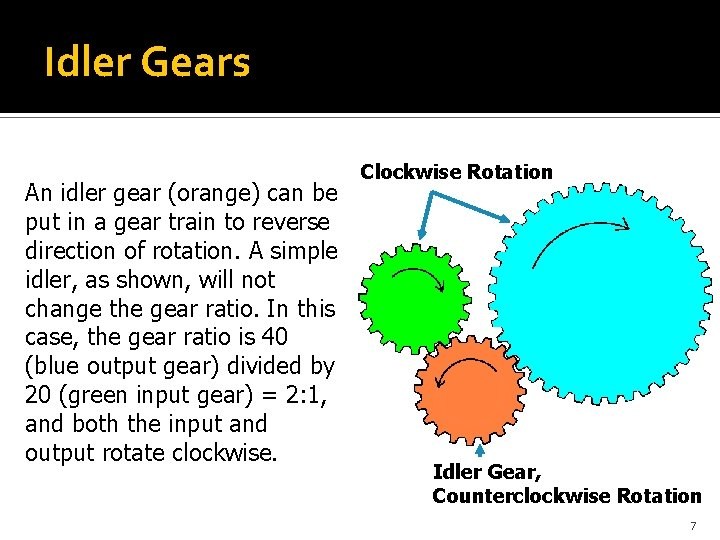Idler Gears An idler gear (orange) can be put in a gear train to reverse direction of rotation. A simple idler, as shown, will not change the gear ratio. In this case, the gear ratio is 40 (blue output gear) divided by 20 (green input gear) = 2: 1, and both the input and output rotate clockwise. Clockwise Rotation Idler Gear, Counterclockwise Rotation 7Gears used to change the direction of power flow Gears have many functions and can be used to change the direction of a power flow. Use the picture to identify direction of motion and types of gears used. 8Multiple parts of a gear Many dimensions are considered when designing a gear. 9Tooth Contact Area Pitch Circle Pitch Line The contact area on a tooth is shown in gray and should be centered on the pitch line. 10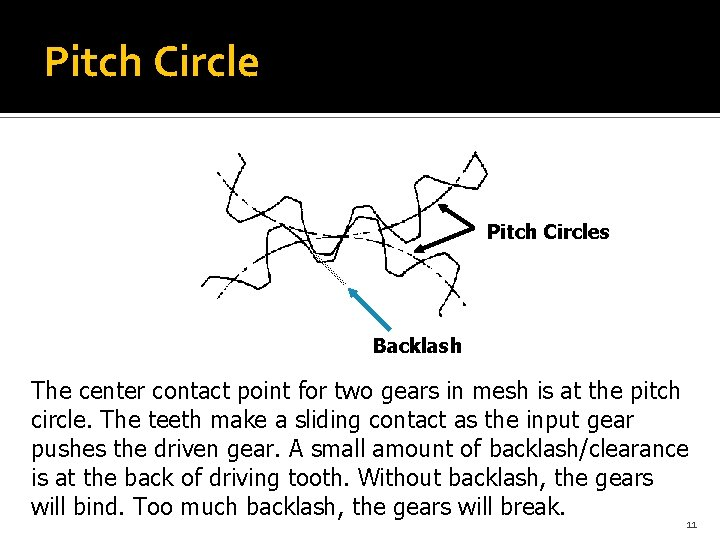Pitch Circles Backlash The center contact point for two gears in mesh is at the pitch circle. The teeth make a sliding contact as the input gear pushes the driven gear. A small amount of backlash/clearance is at the back of driving tooth. Without backlash, the gears will bind. Too much backlash, the gears will break. 11